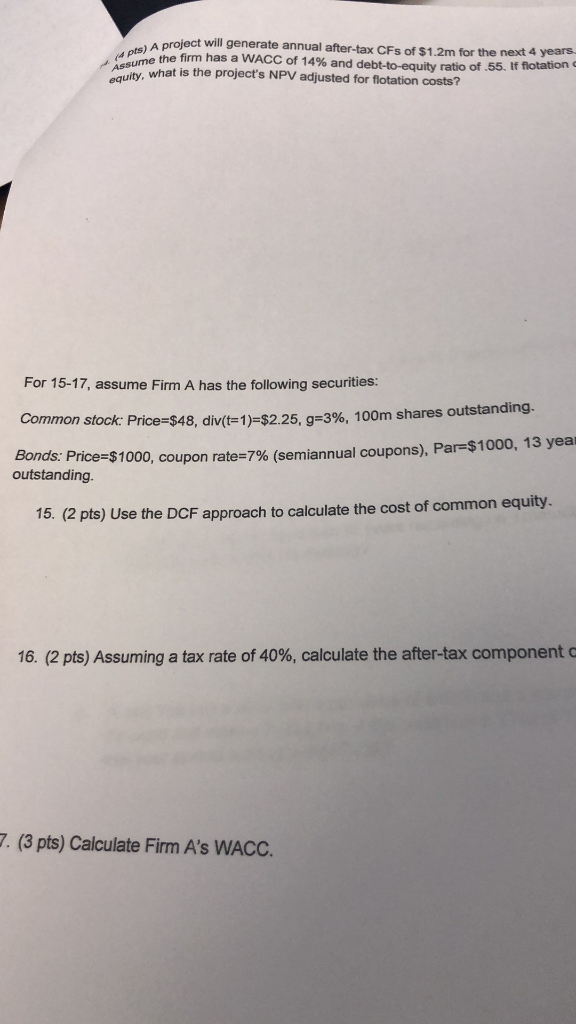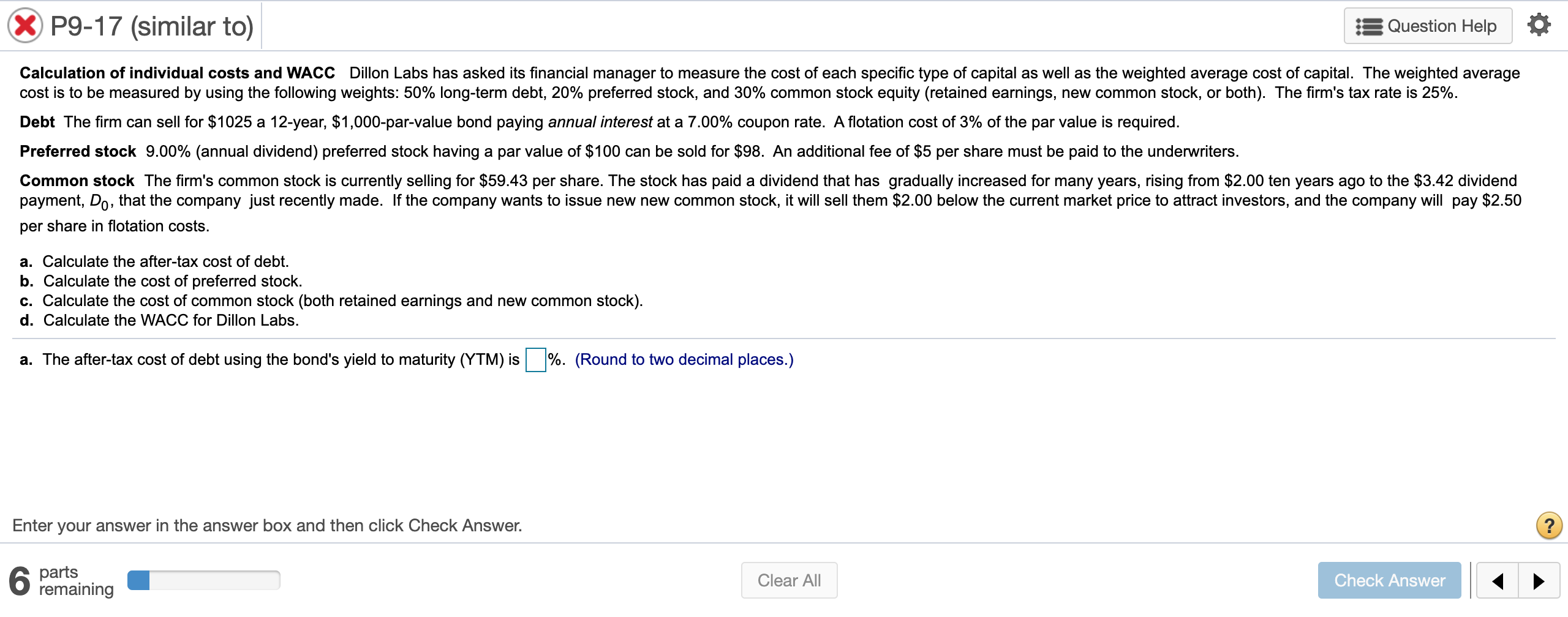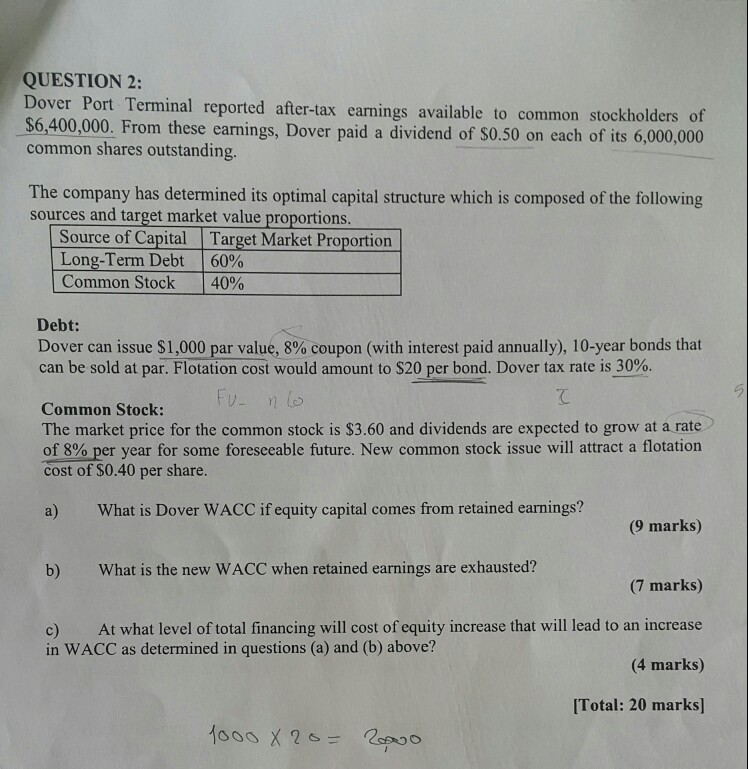# A project will generate annual after-tax CFs of \$1.2m for the next 4 years what is...A project will generate annual after-tax CFs of \$1.2m for the next 4 years what is the project's NPV adjusted for flotation costs? ne the firm has a WACC of 14% and debt-to-equity ratio of .55. If flotation c equity qulity, For 15-17, assume Firm A has the following securities: Common stock: Price-s 48, div t-1)-\$2.25, g-3%, 100m shares outstanding. -7% (semiannual coupons), Par-\$1000, 13 yea Bonds: Price-\$1000, cou outstanding. pon rate 15. (2 pts) Use the DCF approach to calculate the cost of common equity. 16, (2 pts) Assuming a tax rate of 40%, calculate the after-tax component c 7. (3 pts) Calculate Firm A's WACC.

a) Cost of equity, rs = D1 / P + g = 2.25 / 48 + 3% = 7.69%

b) Cost of debt, rd = coupon rate as the price of the bond is \$1,000 = 7%

After-tax cost of debt = rd x (1 - tax) = 7% x (1 - 40%) = 4.20%

c) WACC = wd x rd x (1 - tax) + ws x rs

In order to wd and ws, which are weights of debt and equity, I need the bonds outstanding which are not available.

Value of equity, E = 100 x 48 = 4800 million

If bonds outstanding are 1 million, then D = 1 x 1000 = 1000 million

wd = 1000 / (1000 + 4800) and ws = 4800 / (1000 + 4800)

#### Earn Coin

Coins can be redeemed for fabulous gifts.

Similar Homework Help Questions
• ### A firm is considering a project that will generate perpetual after-tax cash flows of \$17,000 per year beginning next...

A firm is considering a project that will generate perpetual after-tax cash flows of \$17,000 per year beginning next year. The project has the same risk as the firm’s overall operations and must be financed externally. Equity flotation costs 15 percent and debt issues cost 4 percent on an after-tax basis. The firm’s D/E ratio is 0.5. What is the most the firm can pay for the project and still earn its required return? (Do not round intermediate calculations. Round...

• ### A firm that is in the 35% tax bracket forecasts that it can retain \$4 million...

A firm that is in the 35% tax bracket forecasts that it can retain \$4 million of new earnings plans to raise new capital in the following proportions: 60% from 30-year bonds with a flotation cost of 4% of face value. Their current bonds are selling at a price of 91 (91% of face value), have 4 years remaining, have an annual coupon of 7%, and their investment bank thinks that new bonds will have a 40 basis point (0.40%)...

• ### a.  The​ after-tax cost of debt using the​ bond's yield to maturity​ (YTM) is The​ after-tax...a.  The​ after-tax cost of debt using the​ bond's yield to maturity​ (YTM) is The​ after-tax cost of debt using the approximation formula is b.  The cost of preferred stock is c.  The cost of retained earnings is The cost of new common stock is d.  Using the cost of retained​ earnings, the​ firm's WACC is Using the cost of new common​ stock, the​ firm's WACC is X P9-17 (similar to) Question Help Calculation of individual costs and WACC Dillon...

• ### Target % in Capital Structure Component Cost (pre-tax) Component Cost (after-tax) Weighted Component Cost Debt 35.00%...

Target % in Capital Structure Component Cost (pre-tax) Component Cost (after-tax) Weighted Component Cost Debt 35.00% Preferred Stock 2.00% Equity 63.00% Tax Rate = 35.00% WACC = Outstanding Bond Preferred Stock Info Common Stock Info (Annual Coupons) Preferred Divided 3 Current Dividend \$2.00 Time to Maturity (years) 10 Current Market Price 50 Current Price \$81.00 Coupon Rate APR 6.00% Preferred Yield 6.00% Expected Growth in Dividends 3.00% Face Value \$1,000.00 Expected Return on Equity 5.54% Current Market Price \$975.00 YTM...

• ### Target % in Capital Structure Component Cost (pre-tax) Component Cost (after-tax) Weighted Component Cost Debt 25.00%...

Target % in Capital Structure Component Cost (pre-tax) Component Cost (after-tax) Weighted Component Cost Debt 25.00% Preferred Stock 8.00% Equity 67.00% Tax Rate = 35.00% WACC = Outstanding Bond Preferred Stock Info Common Stock Info (Annual Coupons) Preferred Divided 2 Current Dividend \$3.00 Time to Maturity (years) 10 Current Market Price 45 Current Price \$81.00 Coupon Rate APR 6.00% Preferred Yield 4.44% Expected Growth in Dividends 3.00% Face Value \$1,000.00 Expected Return on Equity 6.81% Current Market Price \$1,000.00 YTM...

• ### QUESTION 2: Dover Port Terminal reported after-tax earnings available to common stockholders of \$6,400,000. From these...QUESTION 2: Dover Port Terminal reported after-tax earnings available to common stockholders of \$6,400,000. From these earnings, Dover paid a dividend of \$0.50 on each of its 6,000,000 common shares outstanding. The company has determined its optimal capital structure which is composed of the following sources and target market value proportions. Source of Capital Target Market Proportion Long-Term Debt 60% Common Stock 40% Debt: Dover can issue \$1,000 par value, 8% coupon (with interest paid annually), 10-year bonds that can...

• ### A firm that is in the 35% tax bracket forecasts that it can retain \$4 million...

A firm that is in the 35% tax bracket forecasts that it can retain \$4 million of new earnings plans to raise new capital in the following proportions: 60% from 30-year bonds with a flotation cost of 4% of face value. Their current bonds are selling at a price of 91 (91% of face value), have 4 years remaining, have an annual coupon of 7%, and their investment bank thinks that new bonds will have a 40 basis point (0.40%)...

• ### A firm that is in the 35% tax bracket forecasts that it can retain \$3 million...

A firm that is in the 35% tax bracket forecasts that it can retain \$3 million of new earnings plans to raise new capital in the following proportions: 50% from 20-year bonds with a flotation cost of 5% of face value. Their current bonds are selling at a price of 92 (92% of face value), have 5 years remaining, have an annual coupon of 7.2%, and their investment bank thinks that new bonds will have a 50 basis point (0.50%)...

• ### Bronz Snails company hired you as a consultant to estimate the company’s WACC . The firm’s...

Bronz Snails company hired you as a consultant to estimate the company’s WACC . The firm’s target capital structure is 30.5% Debt, 13.1% Preferred stock and 56.4% Common Equity. The Firms noncallable bonds mature in 15years. The bonds have a 9.5% annual coupon rate, a par value of \$1,000 and a market price of \$1,135. Bonds pay coupon payments semi annually. The firm has 200,000 bonds outstanding. The firm has 7%, \$100 par value preferred stocks. There are 1M shares...

• ### A firm is considering a new project which would be similar in terms of risk to...

A firm is considering a new project which would be similar in terms of risk to its existing projects. The firm needs a discount rate for evaluation purposes. The firm has enough cash on hand to provide the necessary equity financing for the project. Also, the firm: - has 1,100,000 common shares outstanding - current price \$12 per share - next year’s dividend expected to be \$1 per share - firm estimates dividends will grow at 5% per year after...